## F.1 General

36.1413GPPBase Station (BS) conformance testingEvolved Universal Terrestrial Radio Access (E-UTRA)Release 17TS

The global in-channel Tx test enables the measurement of all relevant parameters that describe the in-channel quality of the output signal of the TX under test in a single measurement process.

The parameters describing the in-channel quality of a transmitter, however, are not necessarily independent. The algorithm chosen for description inside this annex places particular emphasis on the exclusion of all interdependencies among the parameters.

## F.2.1 Basic principle

The process is based on the comparison of the actual output signal of the TX under test, received by an ideal receiver, with a reference signal, that is generated by the measuring equipment and represents an ideal error free received signal. All signals are represented as equivalent (generally complex) baseband signals.

The description below uses numbers and illustrations as examples. These numbers are taken from frame structure 1 with normal CP length and a transmission bandwidth configuration of NRB = 100. The application of the text below, however, is not restricted to this parameterset.

## F.2.2 Output signal of the TX under test

The output signal of the TX under test is acquired by the measuring equipment and stored for further processsing. It is sampled at a sampling rate of 30.72 Msps and it is named z(ν). In the time domain it comprises at least 1 frame:: z(ν). It is modelled as a signal with the following parameters: demodulated data content, carrier frequency, amplitude and phase for each subcarrier.

## F.2.3 Reference signal

Two types of reference signal are defined:

The reference signal i1(ν) is constructed by the measuring equipment according to the relevant TX specifications, using the following parameters: demodulated data content, nominal carrier frequency, nominal amplitude and phase for each subcarrier. It is represented as a sequence of samples at a sampling rate of 30.72 Msps in the time domain. The structure of the signal is described in the testmodells.

The reference signal i2(ν) is constructed by the measuring equipment according to the relevant TX specifications, using the following parameters: restricted data content: nominal Reference Symbols and the Primary Synchronisation Channel, (all other modulation symbols are set to 0 V), nominal carrier frequency, nominal amplitude and phase for each applicable subcarrier, nominal timing. It is represented as a sequence of samples at a sampling rate of 30.72 Msps in the time domain.

## F.2.4 Measurement results

The measurement results, achieved by the global in channel TX test are the following:

– Carrier Frequency error

– EVM (Error Vector Magnitude)

– Resource Element TX power

– RS TX power (RSTP)

– OFDM Symbol TX power (OSTP)

Other side results are: residual amplitude- and phase response of the TX chain after equalisation.

## F.2.5 Measurement points

Resource element TX power is measured after the FFT as described below. EVM is calculated after the Equalizer (Ampl./ Phase correction). The result of the frequency synchronisation is the frequency offset. It is performed in the pre- and/or post-FFT domain. The FFT window of 2048 samples out of 2194 samples (data +CP) in the time domain is selected in the box CP removal.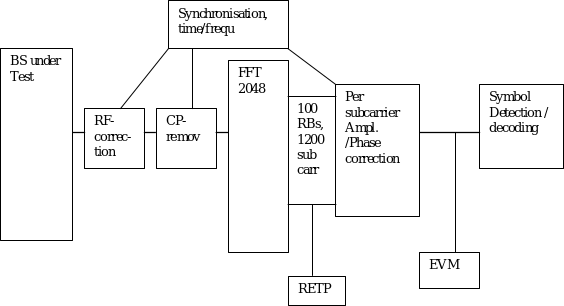Figure E.2.5-1: Measurement points

## F.3.1 Pre FFT minimization process

Sample Timing, Carrier Frequency in z(ν) are varied in order to minimise the difference between z(ν) and i1 (ν) , after the amplitude ratio of z(ν) and i1 (ν) has been scaled. Best fit (minimum difference) is achieved when the RMS difference value between z(ν) and i(ν) is an absolute minimum.

The carrier frequency variation is the measurement result: Carrier Frequency Error.

From the acquired samples one carrier frequency error can be derived.

Note 1: The minimisation process, to derive the RF error can be supported by Post FFT operations. However the minimisation process defined in the pre FFT domain comprises all acquired samples (i.e. it does not exclude the samples inbetween the FFT widths and it does not exclude the bandwidth outside the transmission bandwidth configuration.

Note 2: The algorithm would allow to derive Carrier Frequency error and Sample Frequency error of the TX under test separately. However there are no requirements for Sample Frequeny error. Hence the algorithm models the RF and the sample frequency commonly (not independently). It returns one error and does not distinuish between both.

After this process the samples z(ν) are called z0(ν).

## F.3.2 Timing of the FFT window

The FFT window length is 2048 samples per OFDM symbol. 140 FFTs (286720 samples) cover less than the acquired number of samples (307200 samples in 10 subframes) The position in time for FFT must be determined.

In an ideal signal, the FFT may start at any instant within the cyclic prefix without causing an error. The TX filter, however, reduces the window. The EVM requirements shall be met within a window W<CP. There are three different instants for FFT:

Centre of the reduced window, called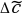, ΔC –W/2 and ΔC +W/2,

The BS shall transmit a signal according to the Test models, intended for EVM. The primary synchronisation signal and the reference signal shall be used to find the centre of the FFT window.

The timing of the measured signal is determined in the pre FFT domain as follows, using z0(ν) and i2(ν) :

1. The measured signal is delay spread by the TX filter. Hence the distinct boarders between the OFDM symbols and between Data and CP are also spread and the timing is not obvious.

2. In the Reference Signal i2(ν) the timing is known.

Correlation between (1.) and (2.) will result in a correlation peak. The meaning of the correlation peak is approx. the “impulse response” of the TX filter.

3. The meaning of “impulse response” assumes that the autocorrelation of the reference signal i2(ν) is a Dirac peak and that the correlation between the reference signal i2(ν) and the data in the measured signal is 0. The correlation peak, (the highest, or in case of more than one highest, the earliest) indicates the timing in the measured signal.

The number of samples, used for FFT is reduced compared to z0(ν). This subset of samples is called z’(ν).

From the acquired samples one timing can be derived.

The timing of the centrewith respect to the different CP length in a slot is as follows: (Frame structure 1, normal CP length)is on Tf=72 within the CP of length 144 (in OFDM symbol 1 to 6)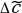is on Tf=88 (=160-72) within the CP of length 160 (in OFDM symbol 0)

## F.3.3 Resource Element TX power

Perform FFT (z’(ν)) with the FFT window timingThe result is called Z’(t,f). The RE TX power is then defined as: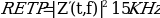From this the Reference Signal Transmit power (RSTP) is derives as follows: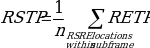,

It is an average power and accumulates the powers of the reference symbols within a sub frame divided by n, the number of reference symbols within a sub frame.

From RETP the OFDM Symbol TX power (OSTP) is derived as follows: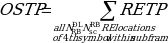It accumulates all sub carrier powers of the 4th OFDM symbol. The 4th (out of 14 OFDM symbols within a subframe (in case of frame type 1 , normal CP length)) contains exclusively PDSCH.

From the acquired samples 10 values for each RSTP and OSTP can be derived.

## F.3.4 Post FFT equalisation

Perform 140 FFTs on z’(ν), one for each OFDM symbol comprising the full frame with the FFT window timing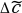. (in case of frame type 1 , normal CP length) The result is an array of samples, 140 in the time axis t times 2048 in the frequency axis f.

The equalizer coefficients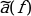and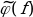are determined as follows:

1. Calculate the complex ratios (amplitude and phase) of the post-FFT acquired signal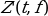and the post-FFT Ideal signal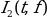, for each reference symbol, over 10 subframes. This process creates a set of complex ratios: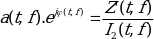2. Perform time averaging at each reference signal subcarrier of the complex ratios, the time-averaging length is 10 subframes. Prior to the averaging of the phases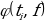an unwrap operation must be performed according to the following definition: The unwrap operation corrects the radian phase angles of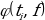by adding multiples of 2*PI when absolute phase jumps between consecutive time instances ti are greater then or equal to the jump tolerance of PI radians. This process creates an average amplitude and phase for each reference signal subcarrier (i.e. every third subcarrier with the exception of the reference subcarrier spacing across the DC subcarrier).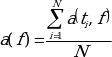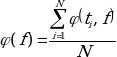Where N is the number of reference symbol time-domain locations ti from Z’(f,t) for each reference signal subcarrier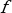.

3. The equalizer coefficients for amplitude and phase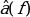and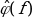at the reference signal subcarriers are obtained by computing the moving average in the frequency domain of the time-averaged reference signal subcarriers, i.e. every third subcarrier. The moving average window size is 19. For reference subcarriers at or near the edge of the channel the window size is reduced accordingly as per figure F. 3.4.

4. Perform linear interpolation from the equalizer coefficients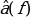and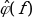to compute coefficients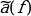,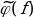for each subcarrier.

The equalized samples are called Z’eq(f,t).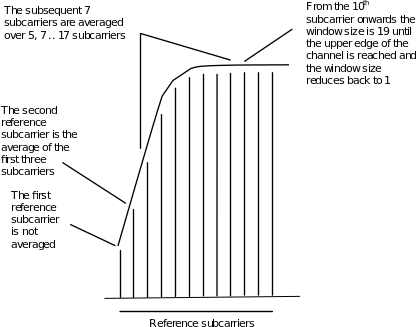Figure F.3.4-1: Reference subcarrier smoothing in the frequency domain

## F.4.1 EVM

For EVM create two sets of Z’eq(f,t)., according to the timing ”ΔC –W/2 and ΔC +W/2”, using the equalizer coefficients from F.3.4.

The equivalent ideal samples are calculated form i1(ν) (clause F.2.3) and are called I(f,t).

The EVM is the difference between the ideal waveform and the measured and equalized waveform.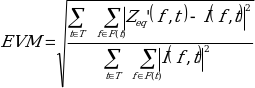,

where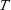is the set of symbols with the considered modulation scheme being active within the subframe or within the sTTI,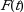is the set of subcarriers within the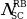resource blocks with the considered modulation scheme being active in symbol t,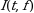is the ideal signal reconstructed by the measurement equipment in accordance with relevant Test models,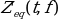is the equalized signal under test.

Note1: Although the basic unit of measurement is one subframe or one sTTI, the equalizer is calculated over the entire 10 subframes measurement period to reduce the impact of noise in the reference symbols.

Note 2: Applicability of EVM calculation:

One EVM value is associated to 12 subcarriers times 1 subframe = pair of 2 RBs = 168 resource elements. For sTTI, one EVM value is associated to 12 subcarriers times 1 slot or 84 resource elements (slot TTI) or times 1 subslot or 24/36 resrouce elements (subslot TTI).

But only a reduced number of REs in this pair of 2 RBs contribute to EVM. Those are the PDSCH or sPDSCH REs, containing the considered modulation scheme. Only those pairs of 2 RBs are evaluated with respect to EVM, which contain the maximum number of PDSCH or sPDSCH REs. (EVM-relevant location in the time/frequency grid ) The others are not evaluated.

In specific:

– For bandwidth 1.4 MHz:

– Only the pairs of 2 RBs containing 138 PDSCH REs are used for EVM. Only those 138 REs contribute to EVM

– All pairs of 2 RBs, which contain less than 138 PDSCH REs, are not evaluated with respect to EVM.

– For all other Bandwidths:

– Only the pairs of 2 RBs containing 150 PDSCH REs are used for EVM. Only those 150 REs contribute to EVM

– All pairs of 2 RBs, which contain less than 150 PDSCH REs, are not evaluated with respect to EVM.

– For sTTI and all Bandwidths:

– Only when EVM value could be evaluated over  sPDSCH REs for slot TTI or [24 or 36] sPDSCH REs for subslot TTI, value is considered for EVM.

– When there are less than  REs for slot TTI or [24 or 36] REs for subslot TTI value is not considered with respect to EVM.

This restriction serves to avoid weighted averaging in F.4.2.

## F.4.2 Averaged EVM

EVM is averaged over all allocated EVM relevant locations in the frequency domain, and 10 consecutive downlink subframes (10 ms):

(The locations in the time-frequency grid are occupied irregularly, see Fig F.4.2-1)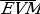is derived by: square the EVM resultsin F.4.1, sum the squares over all EVM relevant locations in the time/frequency grid, divide the sum by the number of EVM relevant locations, square-root the quotient.

The EVM requirements should be tested against the maximum of the average EVM at the window W extremities of the EVM measurements:

Thus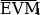is calculated using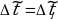in the expressions above and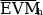is calculated using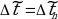. (l and h, low and high. Where l is the timing ΔC –W/2 and and high is the timing ΔC +W/2)

Thus we get: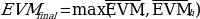For TDD special fields (DwPTS and GP) are not included in the averaging.

15 RBs

10 subframes

Yellow: 136 EVM-relevant locations in the time/frequency grid

Blue: non PDSCH REs

White: RBs with non-maximum number of PDSCH REs

Figure F.4.2-1: Applicability of EVM calculation Example: E-TM1.x, E-TM3.x, 3MHz

### F.4.2.1 Averaged EVM (TDD)

For TDD the averaging in the time domain can be calculated from subframes or slots (for slot TTI) of different frames and should have a minimum of 10 subframes averaging length. TDD special fields (DwPTS and GP) are not included in the averaging.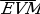frame is derived by: Square the EVM results in a frame. Relevant for EVM are subframes or slots (for slot TTI) in a frame, which are active in the DL, Ndl. Within these subframes or slots, those RBs are relevant, that carry the maximum number of PDSCH REs (same as FDD) or sPDSCH REs (slot TTI). Sum the squares, divide the sum by the number of EVM relevant locations, square-root the quotient. (RMS)

The EVMframe is calculated, using the maximum offrame at the window W extremities. Thus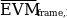is calculated using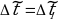and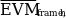is calculated using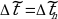. (l and h, low and high. Where l is the timing ΔC –W/2 and and high is the timing ΔC +W/2)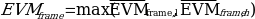In order to unite at least 10 subframes, consider the minimum integer number of radio frames, containing at least 10 EVM relevant subframes or 20 l. Unite by RMS.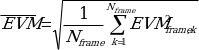,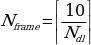The result,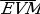, is compared against the limit.

Annex G (informative):
Test Tolerances and Derivation of Test Requirements

The Test Requirements in this specification have been calculated by relaxing the Minimum Requirements of the core specification using the Test Tolerances defined here. When the Test Tolerance is zero, the Test Requirement will be the same as the Minimum Requirement. When the Test Tolerance is non-zero, the Test Requirements will differ from the Minimum Requirements, and the formula used for this relaxation is given in the following tables.

The Test Tolerances are derived from Test System uncertainties, regulatory requirements and criticality to system performance. As a result, the Test Tolerances may sometimes be set to zero.

The test tolerances should not be modified for any reason e.g. to take account of commonly known test system errors (such as mismatch, cable loss, etc.).

Note that a formula for applying Test Tolerances is provided for all tests, even those with a test tolerance of zero. This is necessary in the case where the Test System uncertainty is greater than that allowed in clause 4.1.2. In this event, the excess error shall be subtracted from the defined test tolerance in order to generate the correct tightened Test Requirements as defined in this Annex.

[FFS: For example, a Test System having 0.9 dB uncertainty for test 6.2 Base Station maximum output power (which is 0.2 dB above the limit specified in clause 4.1.2) would subtract 0.2 dB from the Test Tolerance of 0.7 dB defined in this Annex. This new test tolerance of 0.5 dB would then be applied to the Minimum Requirement using the formula defined in Table G.2-1 to give a new range of 2.5 dB of the manufacturer’s rated output power.

Using this same approach for the case where a test had a test tolerance of 0 dB, an excess error of 0.2 dB would result in a modified test tolerance of -0.2 dB.]

Unless otherwise stated, the Test Tolerances in this annex apply to the Test System for testing BS that supports E-UTRA or E-UTRA with NB-IoT in-band/guard band operation or NB-IoT standalone operation.